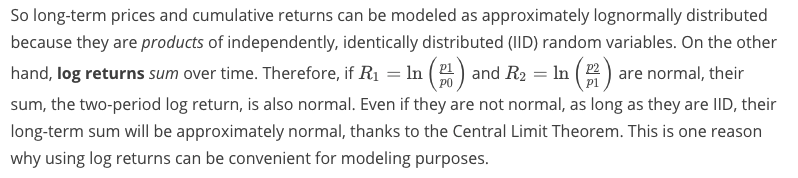AI For Trading: Distributions of Returns and Prices (9)

Distributions of Returns and Prices

Investors are always interested in the potential appreciation or depreciation of financial assets. They'd like to be able to predict what will happen to assets in the future, hence, they'd like to be able to build models of stock prices and returns. An important first step is to think of these prices and returns as random variables, i.e. outcomes of random phenomena, that take on values as described by distributions. Distributions allow us to summarize the behavior of random variables. So, what are the distributions of returns and prices?

One strategy for getting a sense of potential future behavior is to look to the past. Let's look at some data from the stock of a familiar company with a storied past, Apple Inc.

Returns and the Historical Record

Let's look at the adjusted closing price of Apple stock (AAPL) from 1980 up until the present.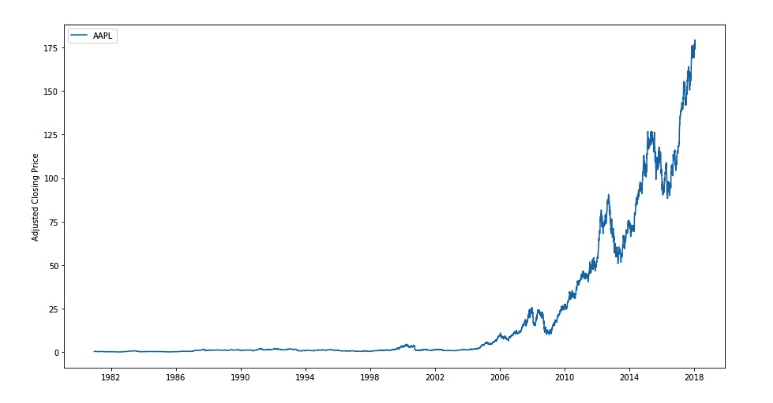If we calculate returns and log returns on these data and plot them, we'll see something like the graphs below.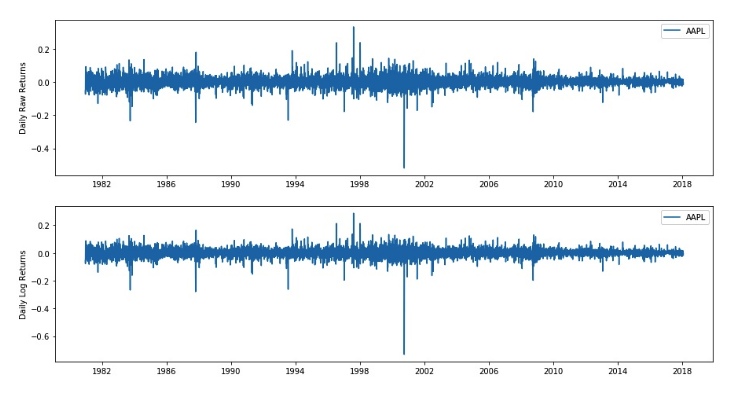The returns and log returns for these daily data have very similar values. This is because daily returns are small—the values are close to 0, so the property \ln(1 + r) \approx rln(1+r)≈r applies. But we're interested in the distribution of these values, so let's look at a histogram.

Normality and Long-Term Investments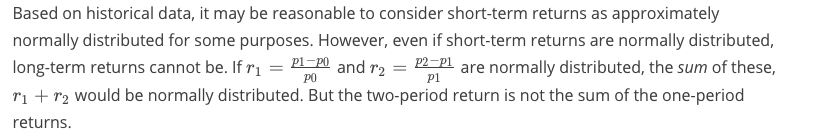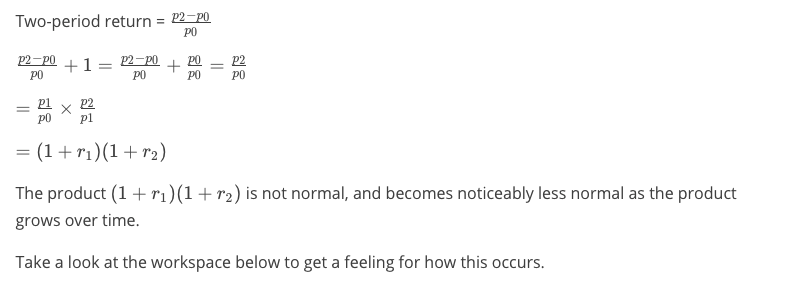Distribution of Log Returns